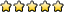## Astronomy C

Luo
Staff EmeritusPosts: 507
Joined: March 21st, 2011, 1:31 pm
State: MN
Has thanked: 0
Been thanked: 13 times

### Re: Astronomy C

Crazy Puny Man wrote:I talked to Luo about this, he said that in the scenario I mentioned I couldn't use the circular velocity formula to calculate the velocity of a star...
By my cursory look at the formulas in question, it seems that people were mixing up formulas for planetary motion (where the mass of the planet can be treated as negligible compared to the mass of the star) with formulas for binary star motion (where the mass of one body cannot necessarily be treated as negligible).
Proud alumnus of Mounds View High School Science Olympiad, Arden Hills, MN
Co-founder of the MIT Science Olympiad Invitational Tournament: http://scioly.mit.edu/

Crazy Puny Man
MemberPosts: 265
Joined: May 25th, 2013, 6:25 pm
State: IN
Has thanked: 0
Been thanked: 0

### Re: Astronomy C

If I remember correctly, the masses I calculated were not disproportionately skewed so that one is much larger than the other.

Syo gave me this link for the full formula: http://en.wikipedia.org/wiki/Orbital_speed, go down to "Taking into account the mass of the orbiting body"

I can't use that formula in this case, since there's not exactly an "orbiting body" in the problem (I got that much)...so why was it like that on the test? Or maybe I'm just remembering something wrong.Also, if you assume that centripetal & gravitational forces are equal (work of syo as well):

Fg=Gm1m2/r^2=Fc=mv^2/r, Fc is the centripetal force towards the center of a circle, Fg is the gravitational force. If you solve for m1 and m2 you get that Gm2/r1^2=v1^2/r1 and Gm1/r2^2=v2^2/r2. That means that v1=sqrt(Gm2/r1) and v2=sqrt(Gm1/r2)

Side note: There's an animation on wikipedia somewhere...ah! here it is:

https://en.wikipedia.org/wiki/Binary_star, scroll down to the section "Astrophysics" and it should be right there.

The stars' velocities increase as their orbits approach the barycenter. If you can imagine that the orbits are circular, would the stars' velocities be constant throughout their orbit? Or would it be something similar to what we're already seeing in the animation?

EDIT: orbital speed link was screwed up

asdfqwerzzz2
MemberPosts: 35
Joined: June 12th, 2013, 7:57 pm
Division: C
Has thanked: 0
Been thanked: 0

### Re: Astronomy C

On the Virginia regional test in the test exchange, there is a question that states, " An imaginary binary system, Rizam, consists of a 10 solar mass star and of a 5.6 solar mass star that orbit with an average distance of 36 AU. Find the orbital period in years." I approached this question by using Kepler's 3rd law, modified by Newton, or M(P^2)=A^3. I Isolated P because I'm solving for orbital period, and that gave me P=sqrt(A^3/M). Here are the steps I took after that:

P=sqrt(36^3/15.6)
P=sqrt(46656/15.6)
P=sqrt(2990.769231)
P≈54.69 years

The answer key states that the orbital period is 26.6 years. Did I make an error somewhere? Or is the answer key incorrect?

havenbro
MemberPosts: 35
Joined: April 9th, 2012, 12:32 pm
Division: C
State: PA
Location: Strath Haven High School
Has thanked: 0
Been thanked: 0

### Re: Astronomy C

I tried your problem using the following equation:

(M1+M2)=A^3/P^2

I found it here: http://www.austincc.edu/jheath/Solar/Ha ... /nvk3l.htm

Now, I'm not an expert in math nor this event, but I did receive the same answer as you, so perhaps the answer key is wrong...?

### Who is online

Users browsing this forum: No registered users and 1 guest